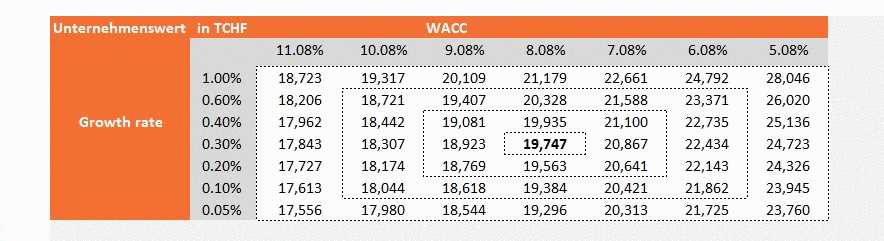Sensitivity analysis shows the effect of changes in input data upon the final result. This analysis shows how sensitive the system is. It gives answers to the following questions:

• What happens to target value when the parameters change their value?
• What value must the input factor have to reach the desired value?
• How do separate input variables influence the project in general – even with inconvenient development?

As opposed to scenario analysis, in this analysis, not more than two factors are changed, so that their effect on the target value is examined (profit or company value) – in scenario analysis several factors are changed simultaneously.

In the following tables, effects of the two input factors (axes) on the target value (values of the table) are presented.Stress testing
In stress testing, input factors are significantly changed, often negatively, so that the target value reaches a minimum value. In general, it is an extreme case. Typically asked questions include the following: How much can a currency grow before the company reports losses? How many losses (and within what period) can happen before the company (without countermeasures) goes bankrupt?